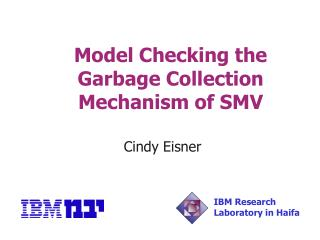DownloadDownload PresentationModel Checking the Garbage Collection Mechanism of SMV

# Model Checking the Garbage Collection Mechanism of SMV

Download Presentation## Model Checking the Garbage Collection Mechanism of SMV

- - - - - - - - - - - - - - - - - - - - - - - - - - - E N D - - - - - - - - - - - - - - - - - - - - - - - - - - -
##### Presentation Transcript

1. Model Checking the Garbage Collection Mechanism of SMV Cindy Eisner IBM Research Laboratory in Haifa

2. Outline • Motivation • Preliminaries • Naïve translation of C to EDL • Radical abstraction • A practical application • The garbage collection mechanism of SMV • Results • False negatives and false positives • Conclusion

3. Motivation • Model checking hardware is “old hat” • IBM has an industrial strength model checker for hardware • Software is the next frontier • Question: just how far can we go with the existing hardware model checker?

4. Preliminaries • RuleBase – IBM’s hardware model checker • VHDL/Verilog hardware design • EDL environment • Sugar specification • For software: • C program (translate to EDL) • EDL behavior of inputs • Sugar specification

5. Preliminaries (EDL) • EDL is a dialect of SMV var a, b, c: boolean; assign next(a) := b & c; define d := a | c;

6. Preliminaries (Sugar) • Sugar is syntactic sugaring of CTL + regular expressions • One kind of Sugar formula is used in this work: suffix implication Sugar: {true[*],a,b[*],c[+]}(d) CTL: EF(a  EX E[b U E[c U (c & d)]])

7. Preliminaries (cont.) • Basic idea: • tool c2edl translates C to EDL as shown on next slides • write properties by hand in Sugar • run RuleBase – see how far we get

8. getmax () { int max, a; 0 a = max = 0; 1 do { 2 if (a > max) 3 max = a; 4 a = input(); 5 } while (a); 6 return(max); } next(a) = if pc=0 then 0 else if pc=4 then {0,1,2,3} else a next(max) = if pc=0 then 0 else if pc=3 then a else max next(pc) = if pc=0 then 1 else if pc=1 then 2 else if pc=2 then if a > max then 3 else 4 … Naïve translation of C to EDL

9. Naïve translation of C to EDL (cont.) • Problems: • Data ranges need to be severely restricted • Pointers • Function calls, including recursion

10. f() { … if (a>b) g(); c = d; } g() { if … … return; } next(pc) = if … else if pc=5 then if (a>b) then 6 else 8 else if pc=6 then 9 else if pc=7 then 8 … else if pc=11 then stack[stackp]; next(stackp) := if pc=6 then stackp+1 else if pc=11 then stackp-1 else stackp Naïve translation to EDL (function calls) f() { … 5 if (a>b) { 6 /*pushcall*/ 7 g(); } 8 c = d; } g() { 9 if (…) 10 … 11 return; }

11. A radical abstraction • Eliminate all variables except for: • program counter • finite stack • Replace variable references with non-deterministic choice: • if (a>b) -> if {0,1}

12. A practical application • For most programs, the abstraction of the previous slide takes away too much • Is there an application for which this approach is practical? • Yes, at least one – the garbage collection mechanism of SMV

13. The garbage collection mechanism of SMV • save_bdd() • release_bdd() • mygarbage() • Example: a = save_bdd(and_bdd(b,c)); d = save_bdd(or_bdd(e,f)); mygarbage(); g = or_bdd(a,d);

14. The garbage collection mechanism of SMV (cont.) • Dangling reference: {true[*], assign_v call_save_bdd_v, (assign_v  call_save_bdd_v)[*], call_mygarbage, assign_v[*], use_v} (false) • All propositions can be expressed as a function of the program counter

15. The garbage collection mechanism of SMV (cont.) • Memory leak: {true[*], call_save_bdd_v, call_release_bdd_v[*], assign_v} (false)

16. Results • Run time of a few hours per group of 20 variables checked • No bugs found in SMV version r2.4.4 • Eight real bugs found in HRL version of SMV under development

17. b = save_bdd(a); c = b; mygarbage(); d = c; … Many could be eliminated through adherence to coding conventions and/or more precise Sugar specifications False negatives

18. False positives • Because depth of stack was limited, possibly false positives as well • It is probably possible to show that because of the extent of the non-determinism, some finite depth is sufficient

19. Conclusion • Our motivation was to see how useful IBM’s existing model checker would be for software • Results were surprising – eight quite sophisticated bugs in real code were found easily • Currently applying hardware model checker to a distributed program with no recursion but without abstracting away the variables